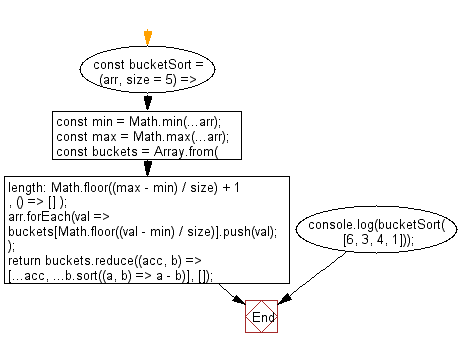# JavaScript Sorting Algorithm: Sort an array of numbers, using the bucket sort algorithm

## JavaScript Sorting Algorithm: Exercise-15 with Solution

Write a JavaScript program to sort an array of numbers, using the bucket sort algorithm.

• Use Math.min(), Math.max() and the spread operator (...) to find the minimum and maximum values of the given array.
• Use Array.from() and Math.floor() to create the appropriate number of buckets (empty arrays).
• Use Array.prototype.forEach() to populate each bucket with the appropriate elements from the array.
• Use Array.prototype.reduce(), the spread operator (...) and Array.prototype.sort() to sort each bucket and append it to the result.

Sample Solution:

JavaScript Code:

``````//Source:https://bit.ly/3hEZdCl
// BucketSort
const bucketSort = (arr, size = 5) => {
const min = Math.min(...arr);
const max = Math.max(...arr);
const buckets = Array.from(
{ length: Math.floor((max - min) / size) + 1 },
() => []
);
arr.forEach(val => {
buckets[Math.floor((val - min) / size)].push(val);
});
return buckets.reduce((acc, b) => [...acc, ...b.sort((a, b) => a - b)], []);
};
console.log(bucketSort([6, 3, 4, 1]));
```
```

Sample Output:

```[1,3,4,6]
```

Flowchart:Live Demo:

See the Pen javascript-common-editor by w3resource (@w3resource) on CodePen.

Improve this sample solution and post your code through Disqus

What is the difficulty level of this exercise?

Test your Programming skills with w3resource's quiz.

﻿